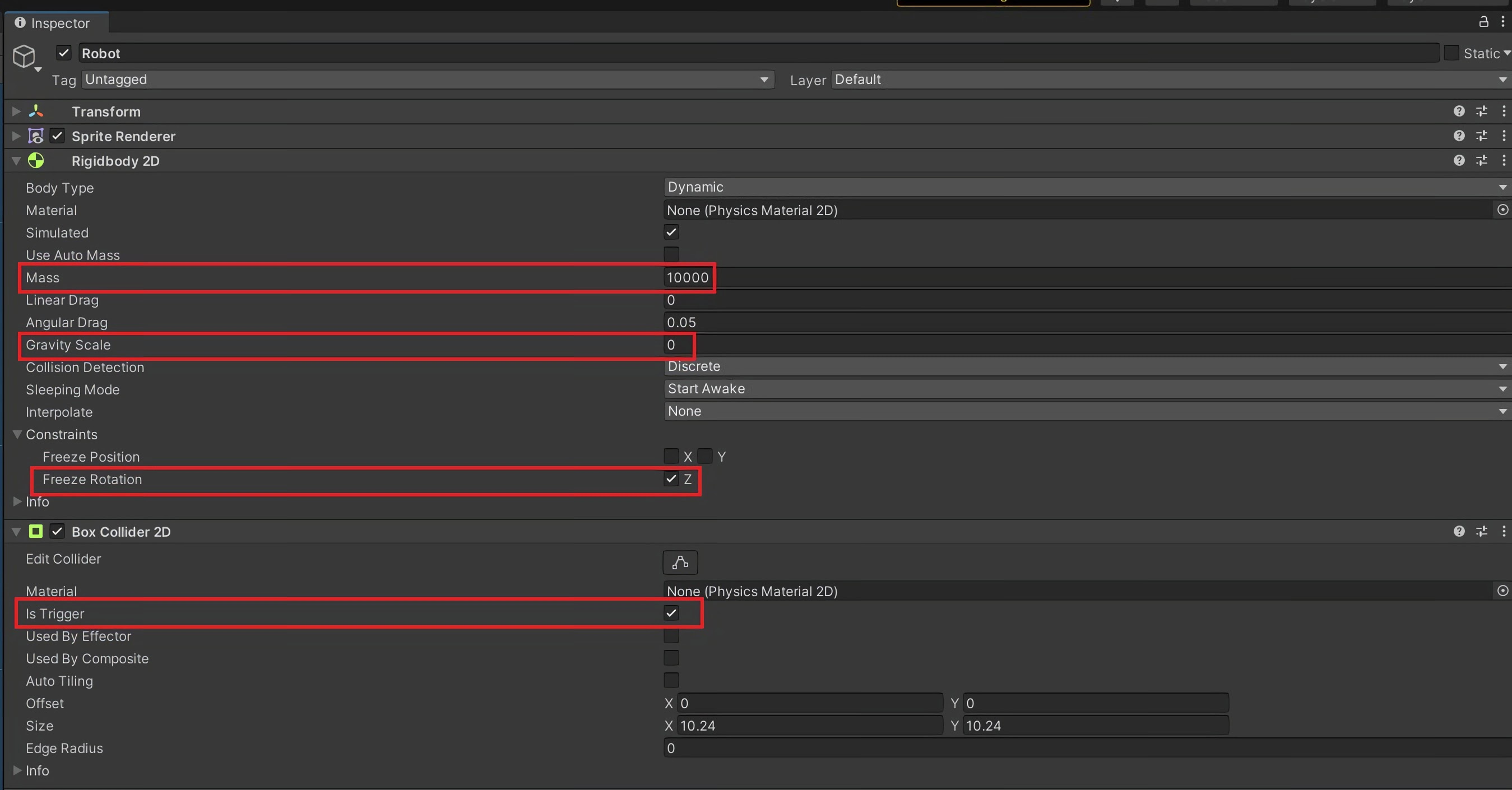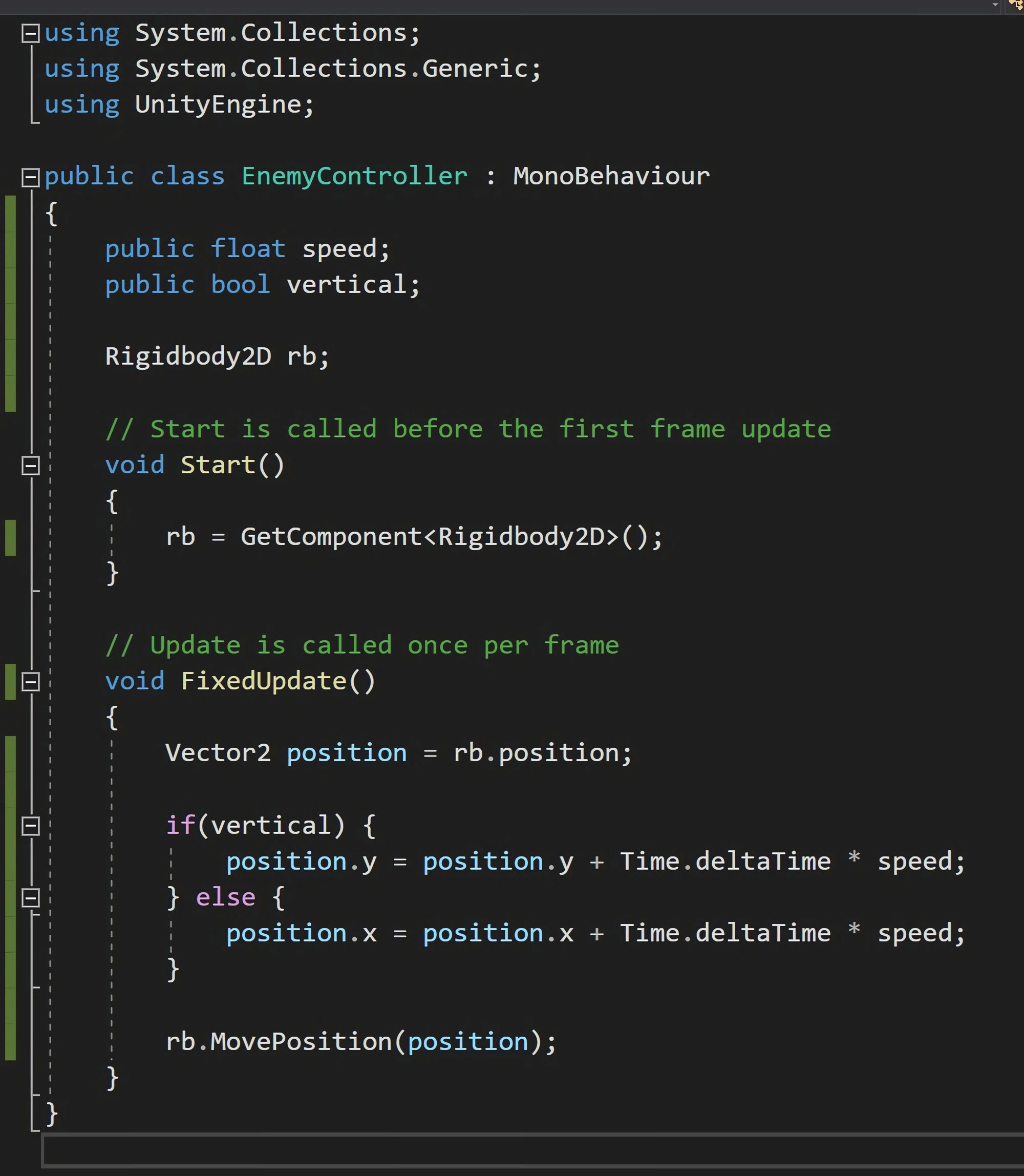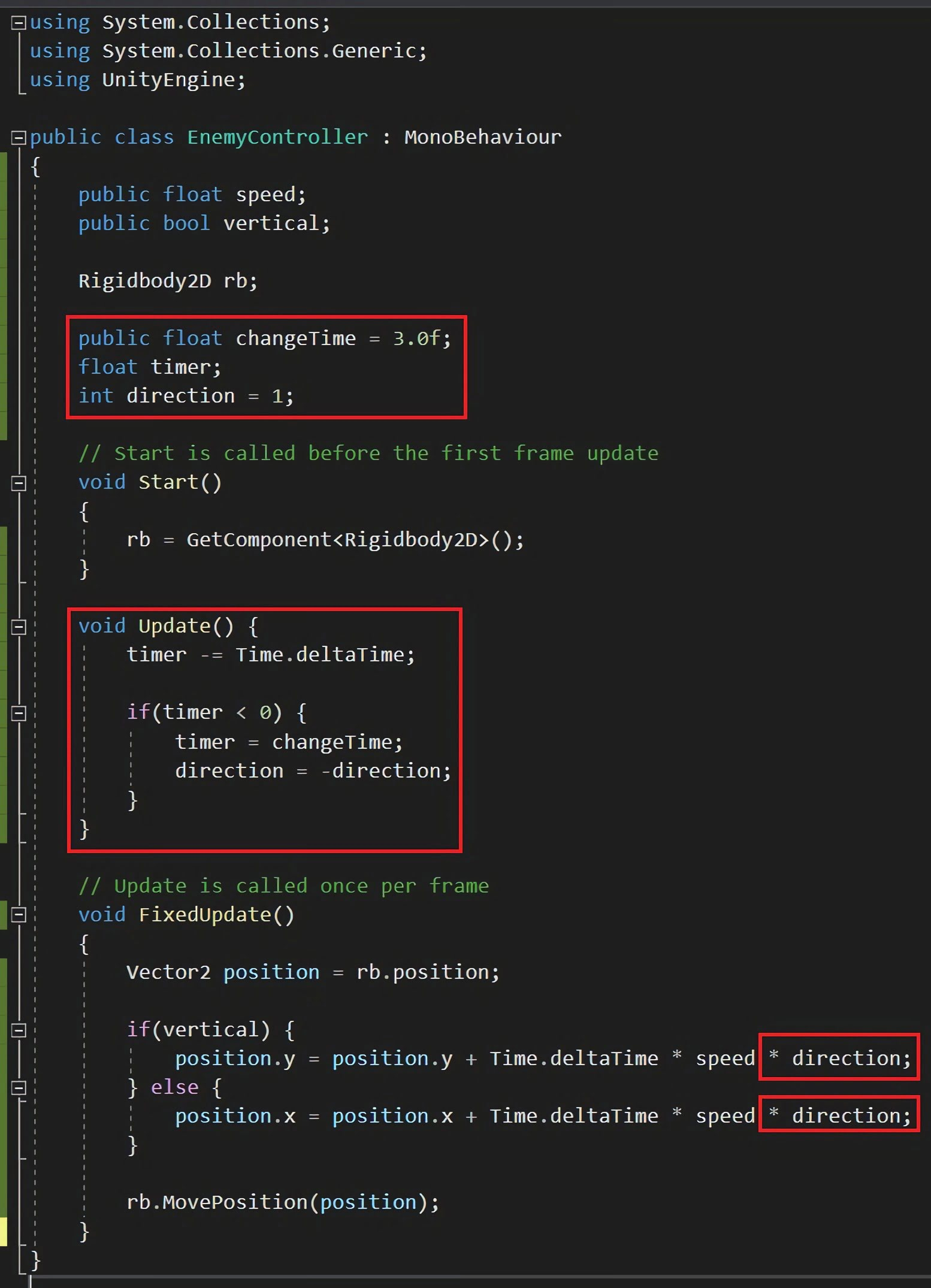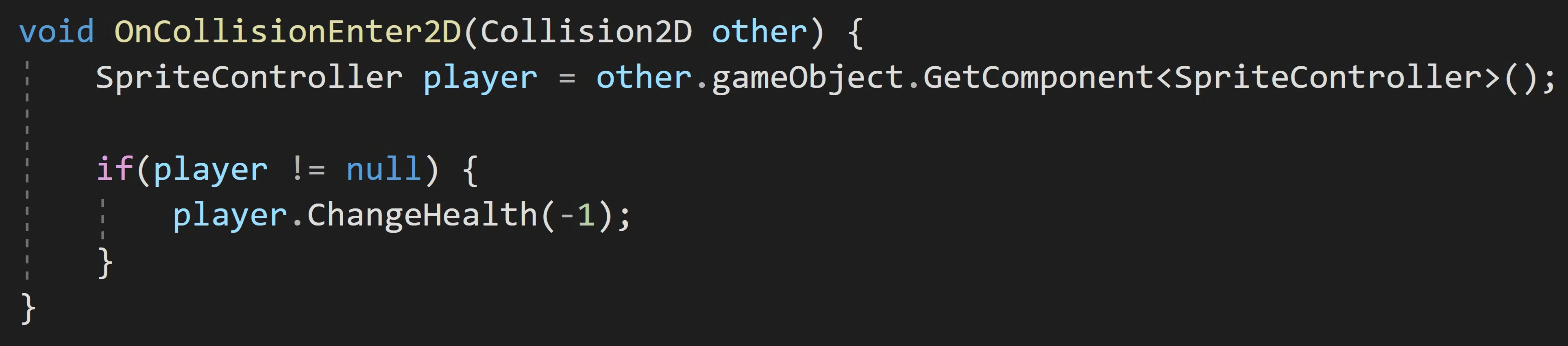# Gaming in Unity

### 13. Unity 2D Game 9 - RobotIt's time to add a Robot that will patrol the area!

### Step 1 - Robot Script - Movement

Import Robot.png into Assets -> Characters.

Place the Robot into the Hierarchy and scale it to your desired size.

Add a Rigidbody 2D and Box Collider 2D.

Set gravity scale to 0 and constrain Z rotation. I've also set mass to 10,000 so Robot can't be pushed around by the main character.

Edit the Box Collider.Next, we need to create a script to control the Robot (make it go back and forth + up and down).

Go to Assets -> Scripts and create a script called EnemyController.

Create a speed and a RigidBody2d variable:

``````public float speed;
Rigidbody2D rb;
``````

Add a boolean called vertical so the Robot can move up and down.

``````public bool vertical;
``````

In void Start(), get the Rigidbody2D component:

``````rb = GetComponent<Rigidbody2D>();
``````

In void FixedUpdate(), add code to change the rigidbody's position. This code checks if the Robot is moving vertically and then decides where to change the X or Y position:

``````Vector2 position = rb.position;

if (vertical) {
position.y = position.y + Time.deltaTime * speed;
} else {
position.x = position.x + Time.deltaTime * speed;
}

rb.MovePosition(position);
``````### Step 2 - Robot Script - Timer

Next, we'll add a Timer so the Robot changes direction.

Add a few variables to store the time before the Robot's direction changes as well as the Robot's direction:

``````public float changeTime = 3.0f;
float timer;
int direction = 1;
``````

In void Start(), set timer to changeTime:

``````timer = changeTime;
``````

In void Update(), add the following to count the timer:

``````timer -= Time.deltaTime;

if(timer < 0) {
direction = -direction;
timer = changeTime;
}
``````

And now in void fixedUpdate, add * direction to each position calculation.Drag this script onto Robot and set speed to 1 in the inspector.

Press Play and watch Robot walk back and forth!

We can now add damage to the Robot by using similar code found in DamageZone.The Robot can now damage the main character. Don't forget to turn Robot into a prefab by dragging it into your prefab folder.

Created by: David Corish